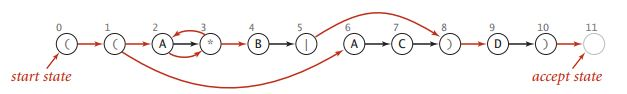# 《算法》笔记 16 - 正则表达式

• 使用正则表达式描述模式
• 非确定有限状态自动机NFA
• 模拟NFA的运行
• NFA的表示
• 构造与正则表达式相对应的NFA
• NFA的模拟与可达性

### 使用正则表达式描述模式

• 连接操作，比如AB，表示由A和B连接而成的模式。
• 或操作，或操作可以在模式中指定多种可能的匹配，用"|"表示。
• 闭包操作，闭包操作可以把模式部分重复任意次数，用"*"表示。
• 括号，括号可以改变默认优先级的顺序。
• 在编程语言中使用的正则表达式还有很多其它语法，但都是基于这几种基本操作的。

### 非确定有限状态自动机NFA具有以下特点：

• 长度为M的正则表达式中的每个字符在所对应的NFA中都有且只有一个对应的状态。NFA的起始状态为0并含有一个虚拟的接受状态M；
• 字符表中的字符所对应的状态都有一条从它指出的边，这条边指向模式中的下一个字符所对应的状态（这种边为黑色）；
• 元字符"(" ")" "|" "*"所对应的状态至少含有一条指出的边（这种边为红色），这些边可能指向其它的任意状态；
• 有些状态有多条指出的边，但一个状态只能有一条指出的黑色边。

• 匹配转换，即文本中的某个字符与模式中的一个字符相对应，此时NFA可以扫过这个字符，并经过黑色的边转换到下一状态；
• ε转换，是指NFA经由红色边转换到了一个状态而不扫描文本中的任何字符。

### 模拟NFA的运行

#### 构造与正则表达式相对应的NFA

• 连接操作
连接操作的实现很容易，状态的匹配之后和字母表中字符的对应关系就是连接操作的实现。

• 括号
遇到正则表达式中的左括号时，将它的索引压入栈中，在遇到右括号时，再从栈中弹出。

• 闭包操作
闭包运算符'*'如果出现在单个字符之后，就在这个字符和'*'之间添加相互指向的两条ε转换；如果出现在右括号之后，就在对应的左括号和'*'之间添加相互指向的两条ε转换。

• 或操作
遇到或操作也会添加两条ε转换，比如(A|B)，一条从左括号所对应的状态指向或操作后面第一个字符B所对应的状态，另一条从或操作符的状态指向右括号对于的状态。将"|"运算符的索引压入栈中，这样在到达右括号时从栈的顶部就可以拿到这个索引了。

public class Regular {
private char[] re;
private Digraph G;
private int M;

public Regular(String regexp) {
Stack<Integer> ops = new Stack<Integer>();
re = regexp.toCharArray();
M = re.length;
G = new Digraph(M + 1);

for (int i = 0; i < M; i++) {
int lp = i;
if (re[i] == '(' || re[i] == '|')
ops.push(i);
else if (re[i] == ')') {
int or = ops.pop();
if (re[or] == '|') {
lp = ops.pop();
} else
lp = or;
}

if (i < M - 1 && re[i + 1] == '*') {
}

if (re[i] == '(' || re[i] == '*' || re[i] == ')')
}
}
}

#### NFA的模拟与可达性

public boolean recognize(String txt) {
Bag<Integer> pc = new Bag<Integer>();
DirectedDFS dfs = new DirectedDFS(G, 0);
for (int v = 0; v < G.V(); v++) {
if (dfs.marked(v))
}

for (int i = 0; i < txt.length(); i++) {
Bag<Integer> match = new Bag<Integer>();
for (int v : pc)
if (v < M)
if (re[v] == txt.charAt(i) || re[v] == '.')
}